Question

# Two blocks of masses M1 and M2 are connected by a massless string that passes over...

Two blocks of masses M1 and M2 are connected by a massless string that passes over a massless pulley as shown in the figure. M2. which has a mass of 13.5 kg, rests on a long ramp of angle θ=15.5°. Friction can be ignored in this problem.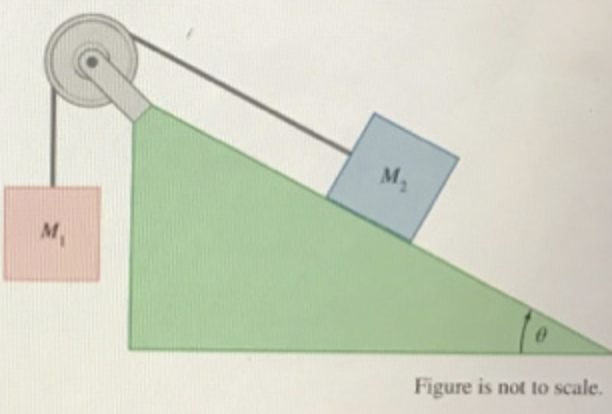Find the value of the mass Mi for which the two blocks are in equilibrium (i.e., not accelerating).

We need at least 10 more requests to produce the answer.

0 / 10 have requested this problem solution

The more requests, the faster the answer.

All students who have requested the answer will be notified once they are available.

#### Earn Coins

Coins can be redeemed for fabulous gifts.

Similar Homework Help Questions
• ### Two blocks of masses M and M2 are connected by a massless string that passes over...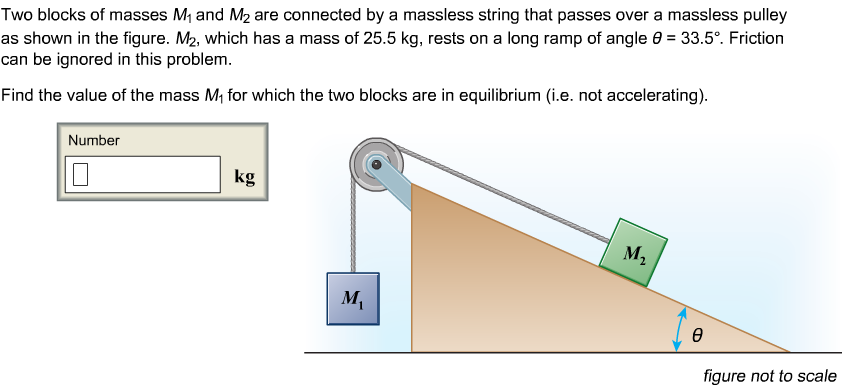Two blocks of masses M and M2 are connected by a massless string that passes over a massless pulley as shown in the figure. M2, which has a mass of 25.5 kg, rests on a long ramp of angle θ-33.5. Friction can be ignored in this problem Find the value of the mass M1 for which the two blocks are in equilibrium (i.e. not accelerating) Number kg figure not to scale

• ### Two blocks with masses M1 and M2 are connected by a massless string that passes over a massless pulley as shown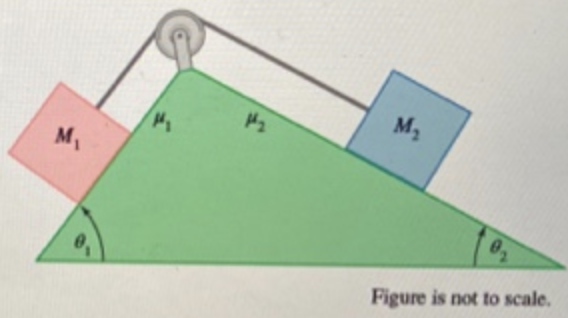Two blocks with masses M1 and M2 are connected by a massless string that passes over a massless pulley as shown. M1 has a mass of 2.25 kg and is on an incline of θ1=43.5° with coefficient of kinetic friction μ1=0.205 . M2 has a mass of 6.15 kg and is on an incline of θ2=35.5° with coefficient of kinetic friction μ2=0.105. The two-block system is in motion with the block of mass M2 sliding down the ramp.Find the magnitude...

• ### Two blocks with masses M1 and M2 are connected by a massless string that passes over...

Two blocks with masses M1 and M2 are connected by a massless string that passes over a massless pulley as shown. M1 has a mass of 2.25 kg and is on an incline of 41.5° with coefficient of kinetic friction μ1 = 0.205. M2 has a mass of 6.25 kg and is on an incline of 31.5° with coefficient of kinetic friction μ2 = 0.105. Find the magnitude of the acceleration of M2 down the incline.

• ### Two blocks of masses Mi and M2 are connected by a massless string that passes over...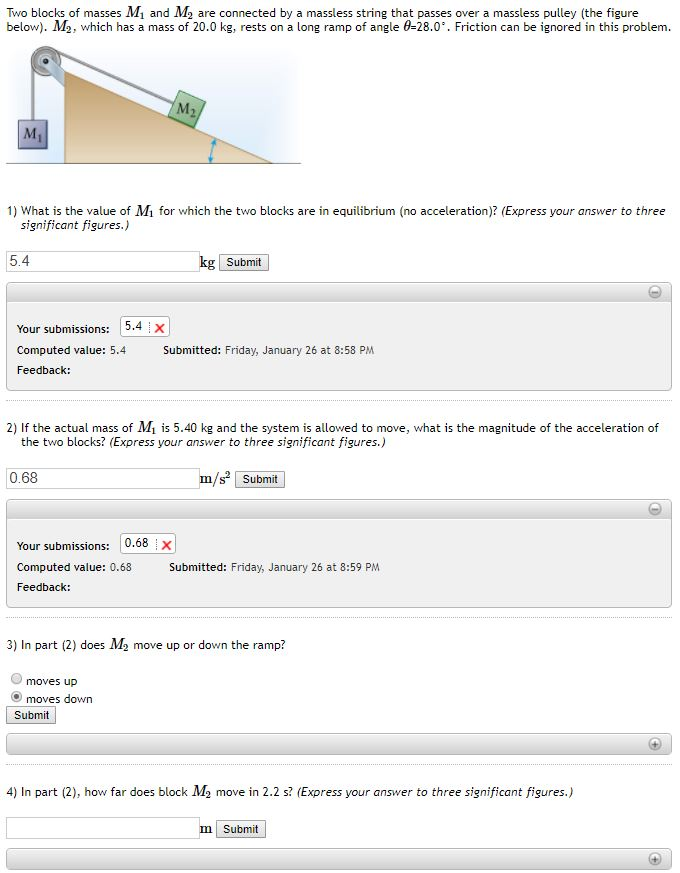Two blocks of masses Mi and M2 are connected by a massless string that passes over a massless pulley (the figure below). M2, which has a mass of 20.0 kg, rests on a long ramp of angle 428.0°. Friction can be ignored in this problem. 1) What is the value of Mi for which the two blocks are in equilibrium (no acceleration)? (Express your answer to three significant figures.) 5.4 Submit Your submissions: 15.4 Computed value: 5.4 Feedback: Submitted: Friday,...

• ### Two blocks, M1M1 and M2M2, are connected by a massless stringthat passes over a massless...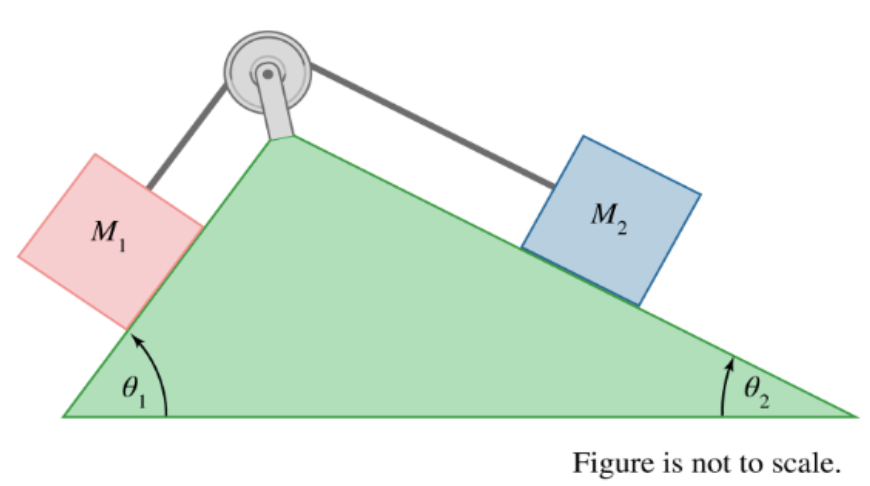Two blocks, M1 and M2, are connected by a massless string that passes over a massless pulley as shown in the figure. M1 has a mass of 7.75 kg and rests on an incline of θ1=73.5° . M2 rests on an incline of θ2=19.5°. Find the mass of block M2 so that the system is in equilibrium (i.e., not accelerating). All surfaces are frictionless.

• ### Two blocks with masses Mi and M2 are connected by a massless string that passes over...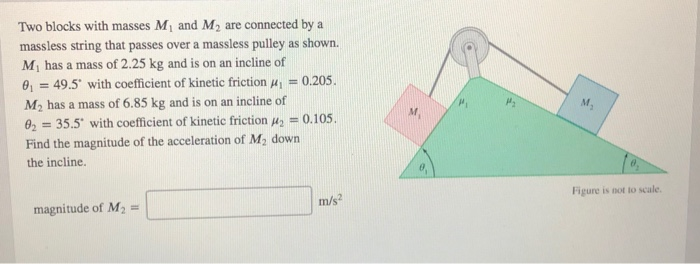Two blocks with masses Mi and M2 are connected by a massless string that passes over a massless pulley as shown. Mi has a mass of 2.25 kg and is on an incline of o, 49.5, with coefficient of kinetic friction μί-0205. M2 has a mass of 6.85 kg and is on an incline of Oz 35.5. with coefficient of kinetic friction μ,-0.105. Find the magnitude of the acceleration of M2 down the incline magnitude of M2 m/s Figure is...

• ### Two blocks with masses m1 and m2 are connected by a massless string over a frictionless...

Two blocks with masses m1 and m2 are connected by a massless string over a frictionless pulley. Block 1 sits on a frictionless horizontal surface and block 2 sits on a plane inclined at an angle θ above the horizontal. The coefficient of friction between block 2 and the incline is µk. The pulley, which is a uniform disk, has a mass mp and a radius R. When you release the blocks, both blocks slide without the string slipping on...

• ### Two blocks of masses m1 and m2 are connected by a light cord that passes over...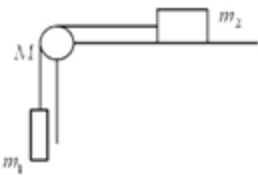Two blocks of masses m1 and m2 are connected by a light cord that passes over a pulley of mass M, as shown. Block m2 slides on a frictionless horizontal surface. The blocks and pulley are initially at rest. When m1 is released, the blocks accelerate and the pulley rotates. The total angular momentum of the system of the two blocks and the pulley relative to the axis of rotation of the pulley isthe same at all times.proportional to I1,...

• ### The two masses "m1" and "m2" shown in the figure connected by a massless string and...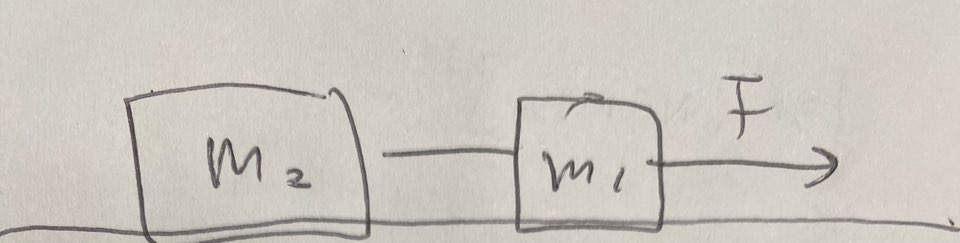The two masses "m1" and "m2" shown in the figure connected by a massless string and are being dropped by a constant horizontal force F a rough horizontal surface. F = 100 N, m1=10 kg, m2=15 kg coefficient kinetic friction between each mass and M_k= 0.2 expression: M2-->M1--> F Questions: 1) Calculate the friction force on M2 2) Calculate the acceleration of the system of the 2 masses 3) Calculate the tension T in the string. H Mz mi

• ### Two blocks with mass m1 = 6.8 kg and m2 = 7.3 kg are connected by a massless string over a frictionless and massless pulley

Two blocks with mass m1 = 6.8 kg and m2 = 7.3 kg are connected by a massless string over a frictionless and massless pulley. The angle of the incline is equal to 32.5°. The kinetic coefficient of friction between m1 and the incline is 0.21. (a)What is the minimum value of the static friction coefficient that will prevent m1 from starting to move if it is at rest. (b)Find the magnitude of the acceleration of the system if m1...